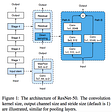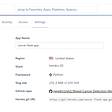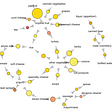# Introduction

Machine learning models are trained by data scientists but too often predictions made are not analyzed to check for bias, fairness and insights. This step is helpful to do what-if analysis on model performance. Google’s what-if tool is very useful in doing this. We need predictions on large sample of test data. In this article I will share simple way for making batch predictions on test data using test dataframe.

# Convert dataframe and predict

The following function is used to convert training data to tf.dataset. We will reuse the same function so that our test data will be in sync with training data.

`def df_to_dataset(dataframe, shuffle=True, batch_size=32):  dataframe = dataframe.copy()  labels = dataframe.pop(target_column)  ds = tf.data.Dataset.from_tensor_slices((dict(dataframe), labels))  if shuffle:    ds = ds.shuffle(buffer_size=len(dataframe))  ds = ds.batch(batch_size)  return ds`

You can reuse the function on test dataframe by adding target_column if your test data does not have it.

`actuals_available = Trueif target_column not in list(test_df.columns):    test_df[target_column] = 0 #any value - we will drop    actuals_available = False`

Now convert dataframe to dataset but make sure shuffling is turned off.

`test_ds = df_to_dataset(test_df, shuffle=False, batch_size=len(test_df))`

Here is the code — please note that predictions should not be shuffled (that is why we turned shuffling off while building dataset)

`test_df[”pred”] = model.predict(test_ds).ravel()`

# Conclusion

Using TF dataset predictions can be made on a large sample of test data. Having both actual value and prediction value in dataframe will help us measure accuracy and do what-if analysis on various facets of data.

## More from Ram Thiruveedhi

Data Science | Machine Learning | Operations Research https://www.linkedin.com/in/ramgit/

Love podcasts or audiobooks? Learn on the go with our new app.

## Fine-Tuning NLP Models With Hugging Face## Becoming a Number in Machine Learning## Tensorflow — day 1+2: High level APIs## FAST AI JOURNEY: COURSE V3. PART 2. LESSON 13.1.## A simple technique to estimate prediction intervals for any regression model## All the Basics You Need to Know About Machine Learning## Using Distributed Machine Learning to Model Big Data Efficiently## Takeaways From 3 Years Working In Machine Learning## Ram Thiruveedhi

Data Science | Machine Learning | Operations Research https://www.linkedin.com/in/ramgit/

## Breast Cancer Prediction using Machine Learning (Part-2)## Building and Deploying a Flask REST API on Heroku(Part 2)## An Introduction to Association Rule Learning## Machine Learning Prerequisite | Python Basics: Functions in python